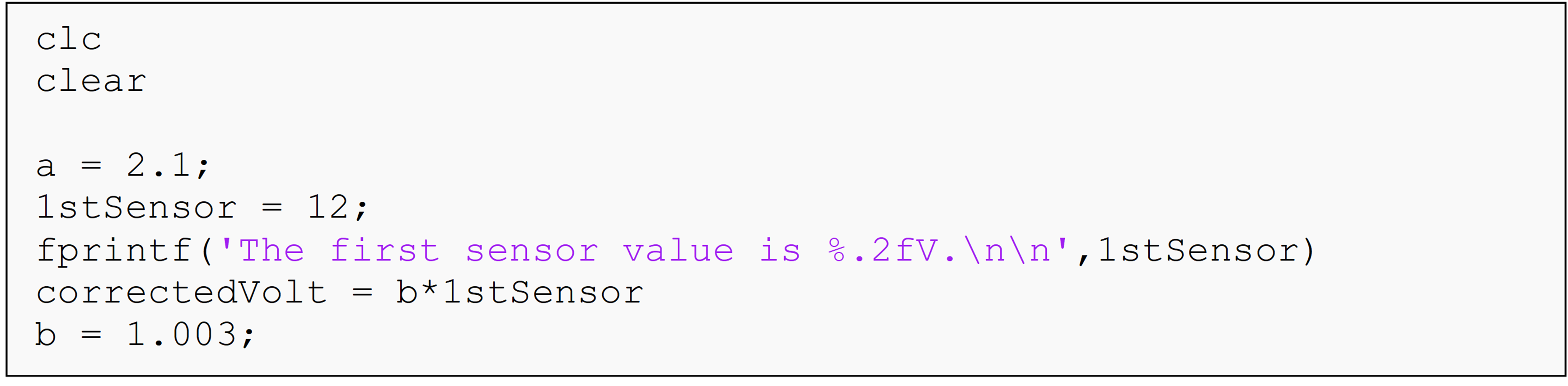# Module 2: BASIC PROGRAMMING FUNDAMENTALS

## Learning Objectives

1. identify problems in your code,

2. interpret errors and warnings,

3. debug an m-file.

Generally, there are two problems you might have with your code: 1) it has an error and will not run, or 2) it does not do what you expect it to do. We will address the first type of problem in this lesson. For the second problem, you will have to complete the rest of the course!

## What is an error?

There are two general types of messages MATLAB may return to alert you to a problem (or potential problem) it sees in your code. These are called error and warning messages, and MATLAB will display them in the Command Window (see Figure 1).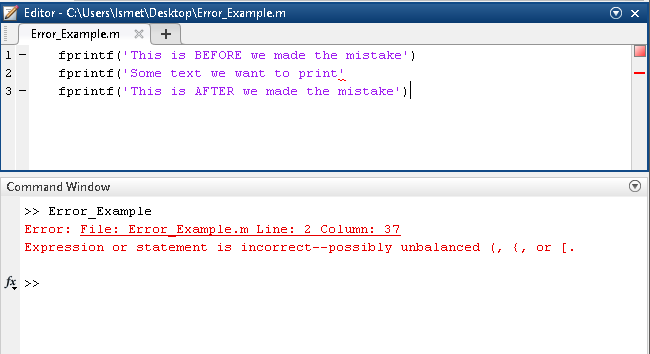Figure 1: An example of an error in MATLAB where it has clearly identified the problem.

Error messages either will not let your program run, or will stop it before it finishes running: it depends on the error. Error messages can identify errors in syntax, problems with the way MATLAB functions are called, etc.

Figure 1 shows an example of an error in MATLAB. As you can see, our program did not execute any lines of code. We know this because the first line of code is written correctly, but it did not display any text to the Command Window. Also, note that MATLAB displays the error message in the Command Window, and tells us exactly what is wrong.

## What is a warning?

A warning is for MATLAB to tell you something about your program without stopping it from running. One example of this is mathematical alerts where MATLAB has checked something behind the scenes and is telling you to be careful (see Figure 2). This is done by the programmers who wrote that MATLAB function to alert you to a specific mathematical problem rather than a programming one. Another example is an outdated or “depreciated” code warning. Computer languages decide to remove functions sometimes and will display “depreciation warnings” that function/syntax will not be supported in future releases of the software (MATLAB in this case).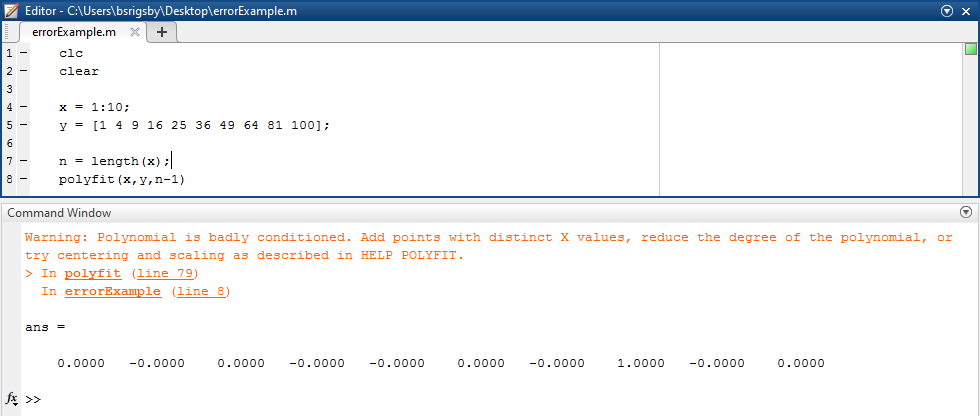Figure 2: An example of a warning in MATLAB where it has identified a potential mathematical problem.

## How can I solve the problem with my code?

One should use a systematic approach to fix, or “debug” a programming problem. The following sub-sections review a general step by step process to identify why your code is broken. There are a variety of errors that can occur in MATLAB; however, the following sections will give you a general way of approach to tackle most errors that you encounter.

Most of the time, if you make an error in the syntax of your program, MATLAB will return an error. Typically, error messages contain at least two types of helpful information. As you can see in Figure 1, it provides a line reference where it believes the problem is occurring (this is often the correct line, but not always). The error message also usually contains some helpful information about the problem, and this information has gotten more and more detailed in newer versions of MATLAB.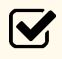Important Note: Always read the error message in full!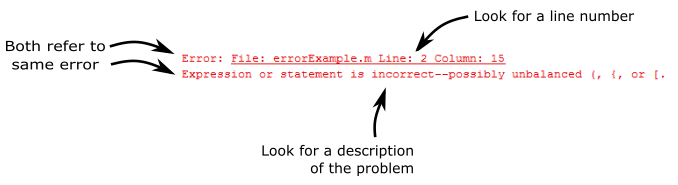Figure 3: Example of how to extract information from an error message.

Once you have gleaned all the information from an error message, then you can move on to further identify and/or fix the issue. There is no exact procedure to follow when debugging, but here are some general steps to try and remember:

1. Check for common causes of errors (often identified in the error message)

1. Line breaks (hitting Enter/Return) in the middle of a function

2. Misspelling (case sensitive) of any used command and function

1. Track usage of variable names in program expressions

2. Ensure that no single variable has more than one name

2. Check code syntax

1. Read the documentation relevant to the commands/functions you are using

2. Check function input order placement (Syntax)

1. Ensure that each function has the correct number and order of inputs

2. Ensure each input is of the correct data type (integer, string, vector, etc.)

3. Think about what is happening in the program!

1. Unsuppress variables to see what values they are taking on.

2. To quickly see all the uses of a variable name in an m-file, click anywhere in the variable name and wait a moment. See Figure 4.

3. It can help to hand-write out the steps the computer will go through. As a rule of thumb, if you do not know how to do something on paper, you do not know how to do it in your program.

4. Check program flowchart, and/or I/O diagram, and/or pseudocode (See Lessons 6.1 and 6.2)

1. This is more helpful when the program does not have errors and still does not return the expected output.
4. Check custom, user-defined functions (See Lesson 7.1)

1. Repeat Steps 1 – 8 for any user-defined functions

2. If there is an error originating from a user-defined function, you can see that in the error message. The m-file where the error occurred is listed in the error message (see Figure 3).

Figure 4 shows an example of the instant find feature of MATLAB. This allows you to click on any variable and have all the instances marked and highlighted. In this case, the variable myVector has been clicked on at line 19 (although you can click on any instance of the variable), and MATLAB has highlighted the other two instances in blue (seen in Figure 4 as grey) on lines 21 and 32.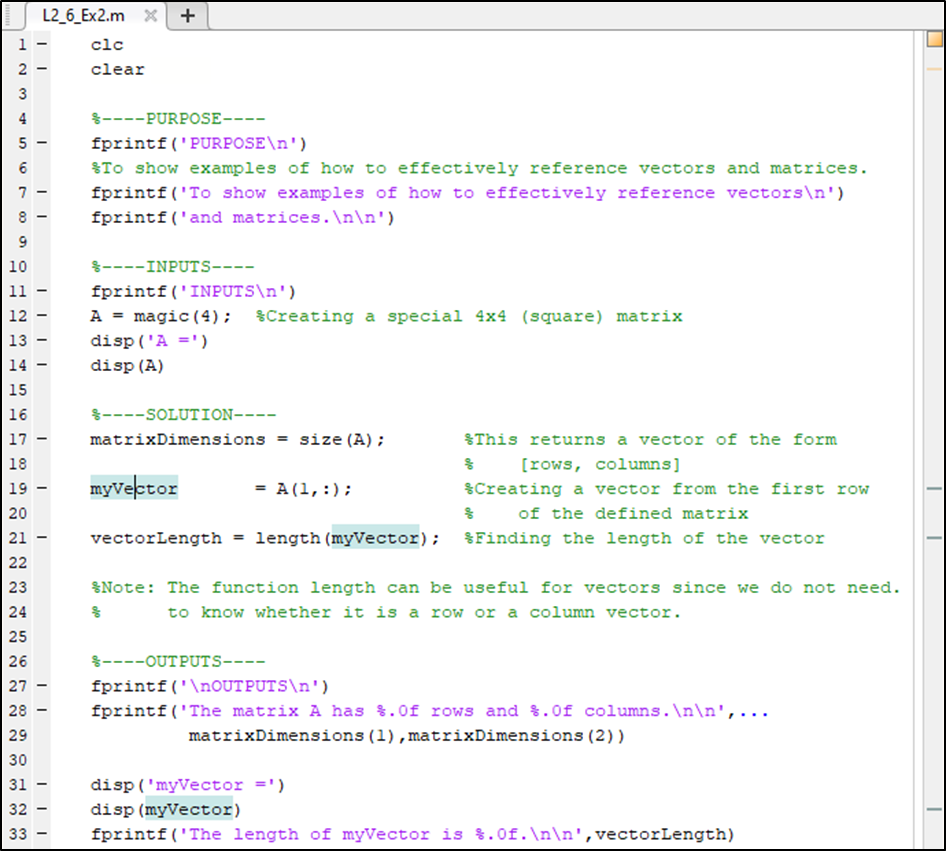Figure 4: m-file showing the find feature.

One can see myVector highlighted in three different places in Figure 4. If your program is longer, you can see that there are grey dashes on the right side of the Editor that show the relative location within the m-file. This makes it a lot easier and faster to check all the different usages of a variable name.

## Can I pause my program part of the way through?

There are two simple ways to pause your program partway through a run. These are breakpoints and the “Pause” button in the Editor tab (Figure 5).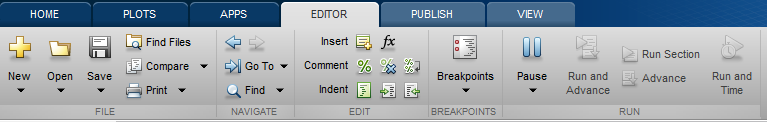Figure 5: Showing the “Pause” button in the Editor tab.

The “Pause” button, once clicked, simply pauses the program wherever it is currently in the execution of the m-file. For example, if Pause is clicked as MATLAB executes line 43, it will pause execution at line 43. If execution is resumed by the programmer (Figure 6), the m-file will continue from line 43.

Breakpoints are a great tool for quickly debugging a program. They function similar to the way the Pause button works except the location where they pause the program execution is precise. Typically, you should favor breakpoints over the Pause button due to the precision of breakpoints.

Figure 7 shows several breakpoints placed in an m-file. Notice the breakpoint circles on lines 17 and 27. Although we used two breakpoints in Figure 7, you can use as many as you like. Placing a breakpoint is done by using the mouse cursor to click in the space to the right of the m-file line number. A breakpoint is denoted with a circle (filled with grey or red color) and can be removed by clicking inside the circle.

When the program execution has been paused, it can be resumed or stopped. The user interface (UI) buttons for this in MATLAB are shown in Figure 6. If “Continue” is selected, the program (m-file) continues as it normally would.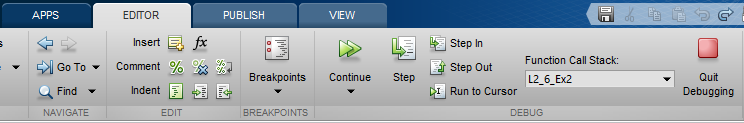Figure 6: Run options when the program has paused.

When an m-file is first executed (use normal execution; i.e., “Run”) with breakpoints, only the segment of code prior to the first breakpoint will run. The m-file will then sequentially execute each segment of code to the next breakpoint for each single execution. After each execution, a green arrow will appear that points to the next executable line of code. Figure 7 shows the indicator arrow (green in MATLAB) and a program m-file which has been partially executed using breakpoints.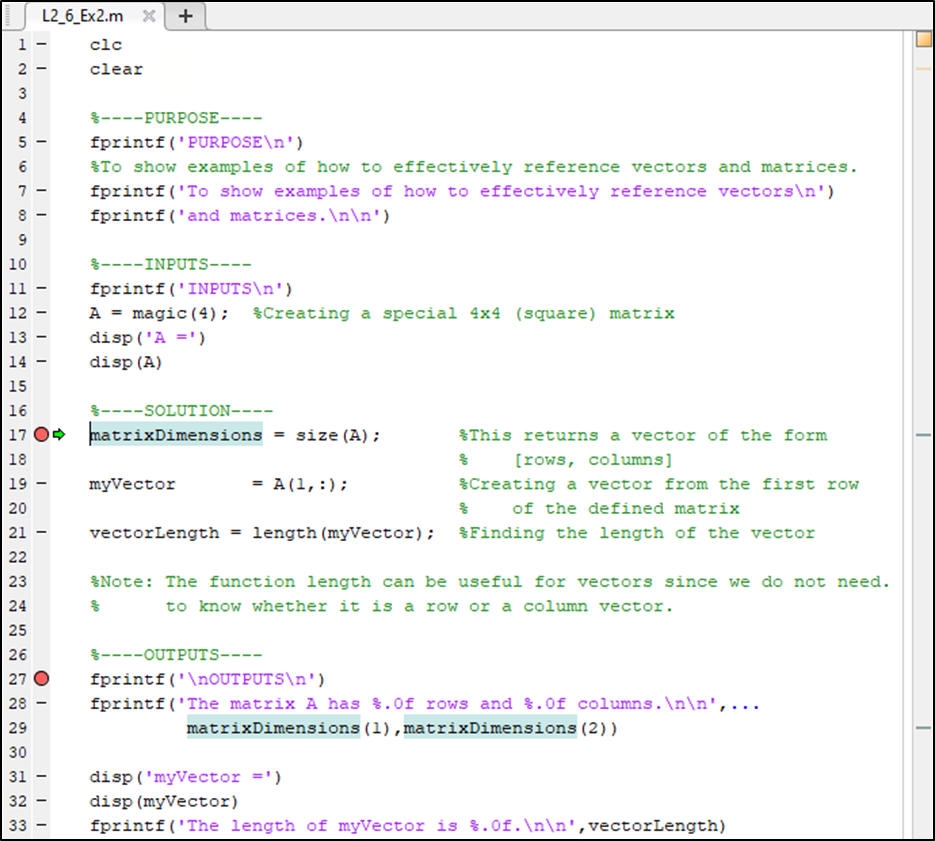Figure 7: Breakpoints placed on an m-file.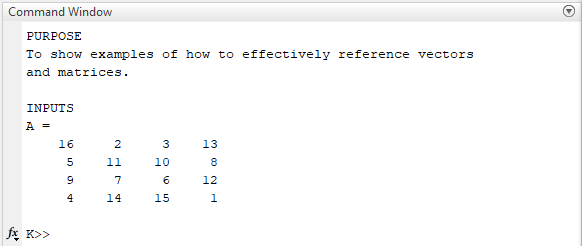Figure 8: Command Window output for code in Figure 6 at the first breakpoint (line 17).

Clicking Run on the m-file shown in Figure 7 will execute lines 1 – 16. It will pause before executing line 17 (and beyond) because we have placed a breakpoint there. Clicking Continue will execute the code from line 17-26. It will pause before line 27 because we have placed a breakpoint there. Finally, clicking Continue the last time will run the rest of the m-file as there are no more breakpoints. Take note of the outputs shown in Figure 8. These are the outputs before the first breakpoint.

## What if I cannot find the exact place of the error?

If you cannot pinpoint the error from an error message or some other information you have gathered, you should try to isolate the problem through other methods. Commenting (%) the part of the code that is nor relevant to the error is a good way to track down the source of the error. You can also make smart use of breakpoints. Remember, MATLAB reads lines of code sequentially, so you should start at the top and work down or vice versa. Unsuppressing (removing the semicolon “;” at the end of a line of code) shows the value of the variable in the Command Window.

## Multiple Choice Quiz

(1). An error given by a MATLAB program means

(a)  the program will continue running, but something is wrong

(b)  something is wrong, and the program will not continue to run

(c)  MATLAB crashed and you need to re-run the program

(d)  you must manually save the program before running it

(2). A good practice while debugging a program is

(a)  unsuppressing a Command Window output

(b)  suppressing a Command Window output

(d)  removing fprintf() statements

(3). The “Pause” button, when pressed at a given line, does the following

(a)  resumes the program from that line

(b)  pauses the program at that line

(c)  stops the whole program

(d)  restarts the program

(4). The number of breakpoints you can use in a program is

(a)  one

(b)  two

(c)  one for each line of code

(d)  ten

(5). A warning given by a MATLAB program implies

(a)  the program has an error

(b)  the program has potential issues that need to be addressed

(c)  the end-user of the program has little to worry about the output of the program

(d)  the program will not run

## Problem Set

Note that you should not expect an error for every “problem”. Errors are returned by MATLAB when there is a problem with syntax, but not necessarily when there is a problem with your solution in general.

(1). Find the problem(s) in the following code and fix them. Then write one paragraph describing the process you used to debug the code. How did you identify the problem(s) and find the solution(s)?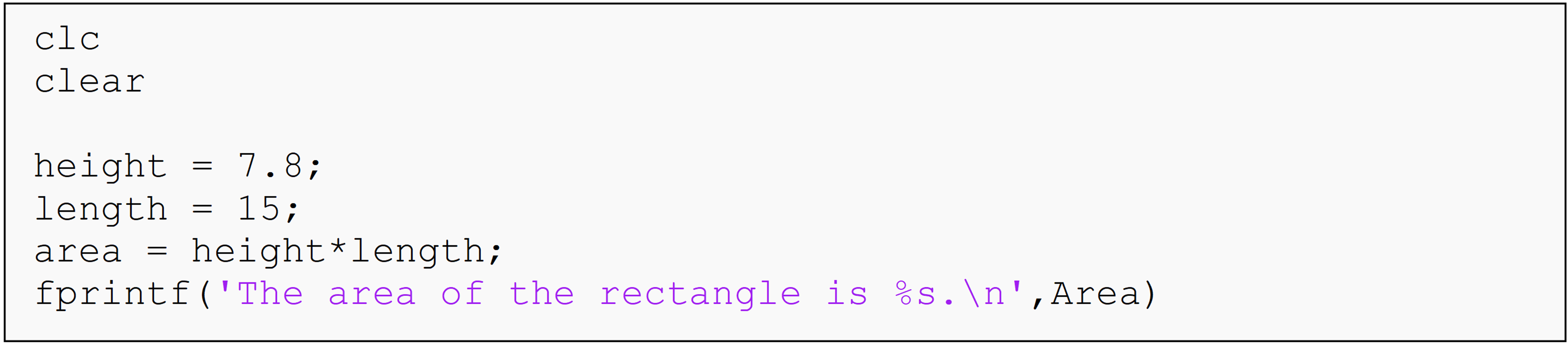(2). Find the problem(s) in the following code and fix them. Then write one paragraph describing what the problem(s) were. Reference the documentation as well.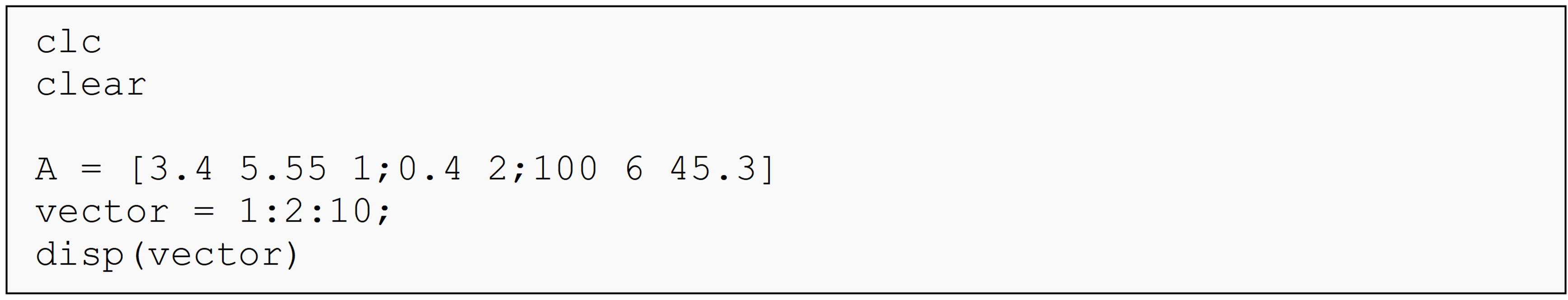(3). Find the problem(s) in the following code and fix them. Then write one paragraph describing what the problem(s) were. Reference the documentation as well.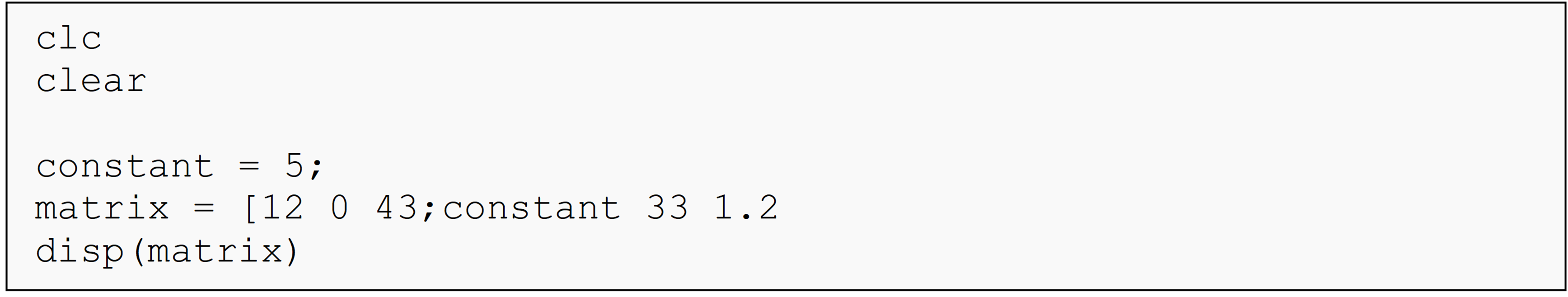(4). Find the problem(s) in the following code and fix them. Then write one paragraph describing what the problem(s) were. Reference the documentation as well.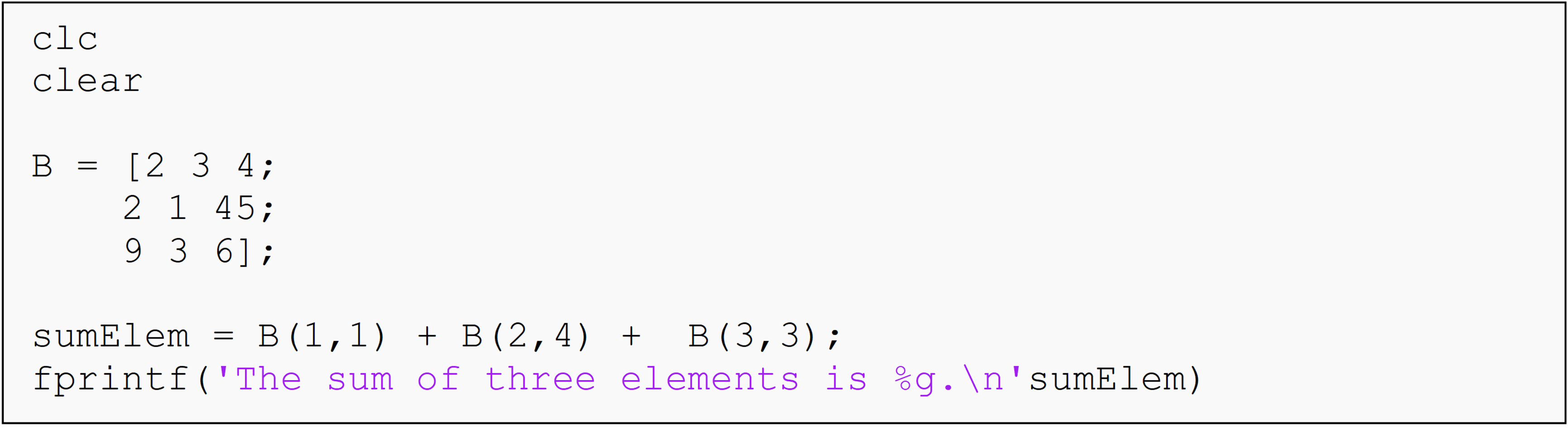(5). Find the problem(s) in the following code and fix them. Then write one paragraph describing what the problem(s) were. Reference the documentation as well.